GeeksforGeeks App
Open AppBrowser
Continue

Valency or Valence of an element is a measure of an atom’s ability to combine with other atoms to create molecules or chemical compounds. The characteristics of an element that indicate how many more atoms can join one of its atoms in a covalent bond are known as valence, or valency, in chemistry. The term, which was first used in 1868, is used to represent both the broad potential of combining an element and the numerical value of the power of combining. Since the majority of bonds are created by the interchange of valence electrons, valence is defined as the number of electrons. The valence electrons determine what valences are and what their meaning is in chemistry.

## What is Valency?

The valency of an atom is equal to the number of valence electrons that this atom can gain or lose during chemical reactions. For example, the amount of hydrogen atoms, chlorine atoms, or double the number of oxygen atoms that one atom of an element may combine with is referred to as its valency.

The valency of an element refers to the number of valence electrons in that element that participates in chemical processes. A chemical compound is created when two or more components are combined in a certain mass proportion. A stable compound is formed when one atom of one element unites with a specific number of atoms of another element. All components have different capabilities for mixing.

## Concept of Valency Explained

The different orbitals (shells) in which electrons are grouped in atoms are denoted by the letters K, L, M, N, and so on. Valence electrons are those found in the outermost orbit or shell of an atom. Because the outermost orbit often contains more energy than the electrons found in other orbits, the valence electrons participate in every chemical reaction.

The Bohr-bury theory predicts that an atom’s outermost orbit will contain up to 8 electrons. However, very little to no chemical activity in the specific element is shown if the outermost orbit is completely filled. Their capacity for merging is reduced to almost nothing.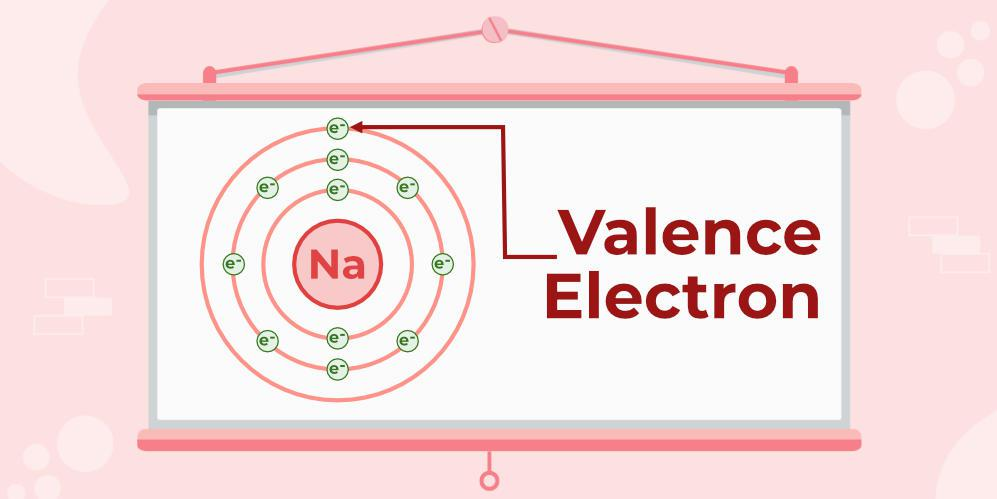Noble gases are least reactive because their outermost orbit is entirely filled, which explains why. The ability of other elements to form noble gas configurations, however, determines how reactive they are. It will also assist in figuring out an atom’s valency.

## How to find the Valency of Elements?

The periodic table shows that all members of the same group of an element have the same valency. If we look at group 8 in the periodic table, all of the elements have achieved octet organization and have entirely occupied their outermost orbit. Therefore, group 8’s elements have no valencies. There are three main ways to determine the valency of any element:

### Octet Rule

The octet rule is applied if valency cannot be determined using the periodic table. According to this principle, atoms of an element or chemical have the propensity to gain or lose electrons in the compound it is present in, giving rise to an increase or decrease in the number of electrons in their outermost orbit. The number of electrons in an atom’s outermost orbit is capped at eight. An atom is considered stable if its outermost shell has 8 electrons.

### Periodic Table

With this approach, the periodic table graphic is used to calculate valency. For instance, all of the metals in column 1 have a valency of +1, including hydrogen, lithium, sodium, and so on. Similar to this, all of the elements in column 17—including fluorine, chlorine, and other elements have valency 1. In column 18, all of the noble gases are organised. These substances have a valency of 0 and are inert.

### Chemical Formulae

The octet rule is the foundation of this method. By studying how they interact chemically with other elements of known valency, it is possible to determine the valencies of several transitional elements or radicals in a given molecule. The octet rule is applied in this situation, where the combining elements and radicals attempt to reach eight electrons in the outermost shell in order to become stable.

Also Check: What is a Chemical Formula?

## Examples of Valency

### Valency of Sodium (Na)

The Atomic number of Na is 11 (K = 2; L = 8; M = 1). They have 1 electron in their outermost shell. So, the valency of Sodium is 1 as shown below: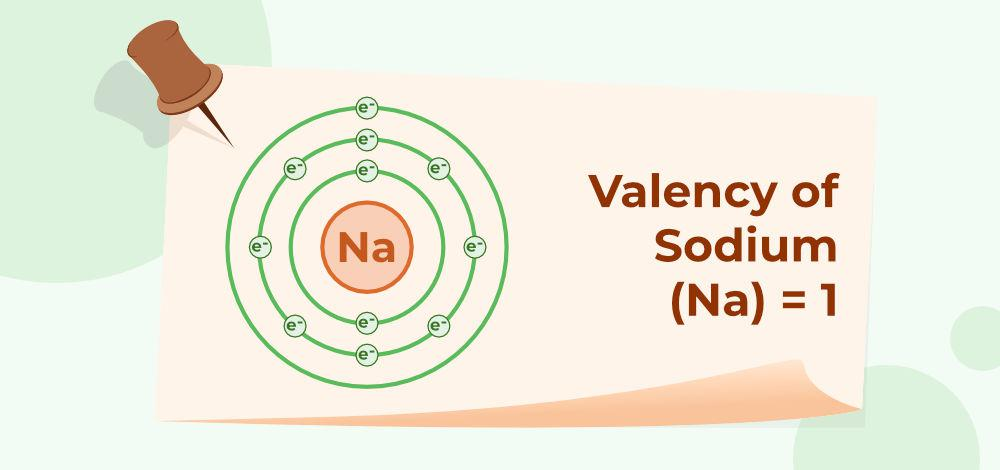### Valency of Carbon (C)

The Atomic number of C is 6 (K = 2; L = 4). They have 4 electrons in their outermost shell. So, the valency of Carbon is 4 as shown below: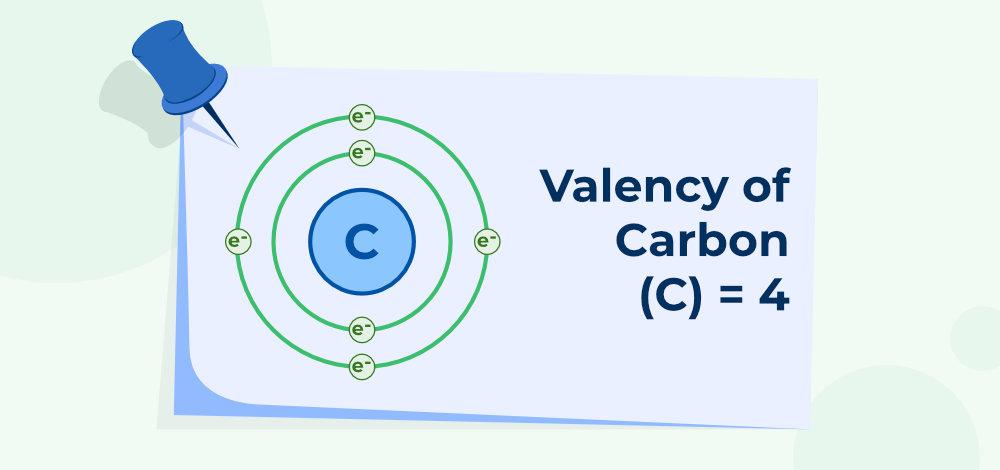### Valency of Oxygen (O)

The Atomic number of O is 8 (K = 2; L = 6; M= 0), They have 6 electrons in their outermost shell. So, the valency of Oxygen is: 8 – 6 = 2 as shown below: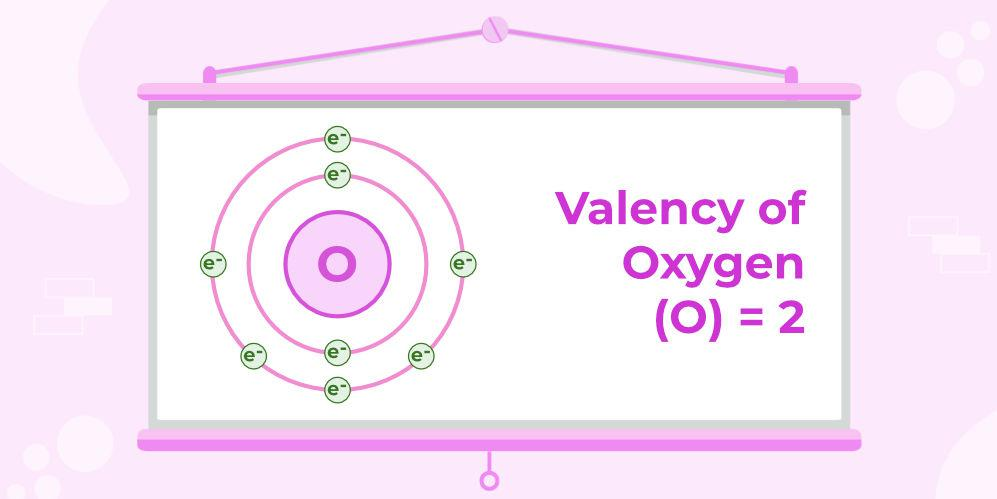### Valency of Nitrogen (N)

The Atomic number of N is 7 (K = 2; L = 5; M= 0). They have 5 electrons in their outermost shell. So, the valency of Nitrogen is: 8 – 5 = 3 as shown below: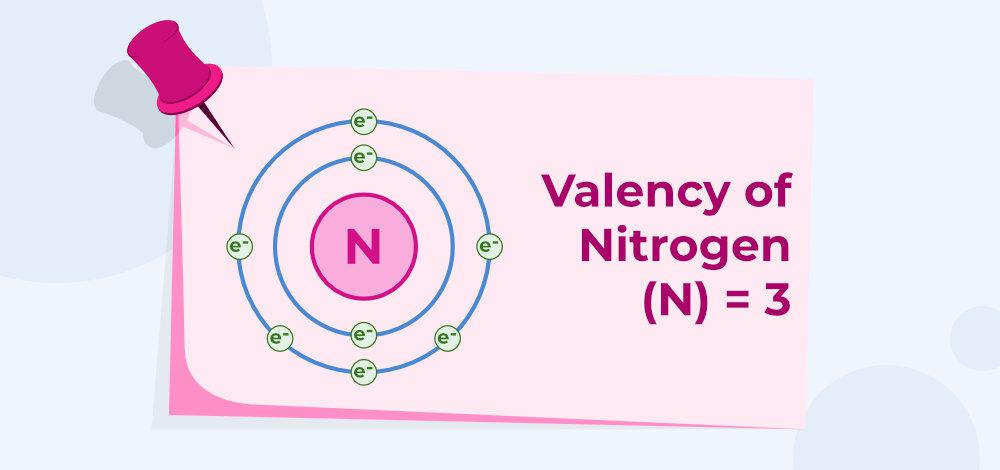### Valency of Sulphur (S)

The Atomic number of S is 16 (K = 2; L = 8; M = 6). They have 6 electrons in their outermost shell. So, the valency of Sulphur is, 8 – 6=2 as shown below: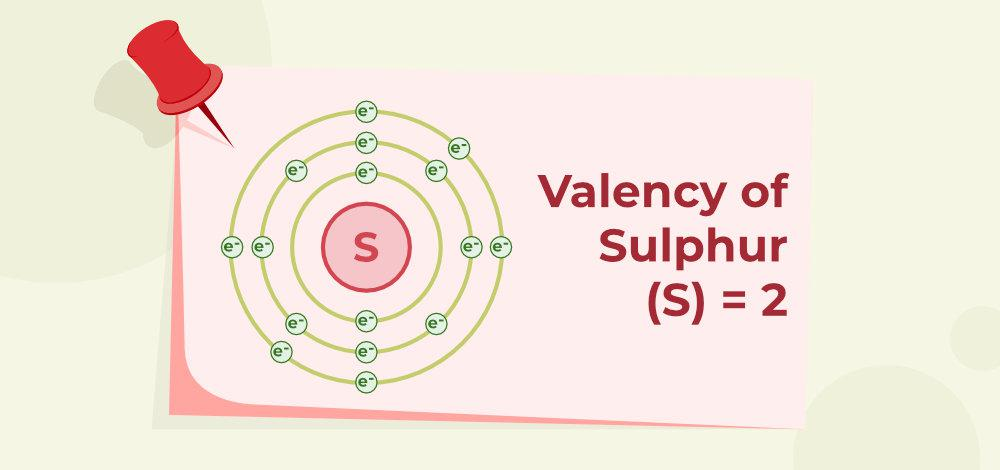### Valency of Chlorine (Cl)

The Atomic number of Cl is 17 (K = 2; L = 8; M = 7). They have 7 electrons in their outermost shell. So, the valency of Chlorine is, 8-7= 1 as shown below: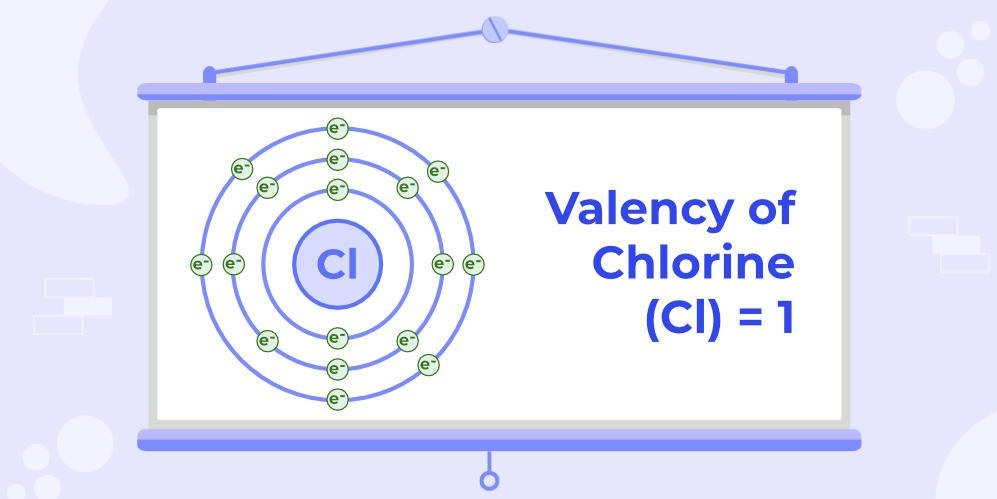### Valency of Aluminium (Al)

The Atomic number of Al is 13 (K = 2; L = 8; M = 3). They have 3 electrons in their outermost shell. So, the valency of Aluminium is 3 as shown below: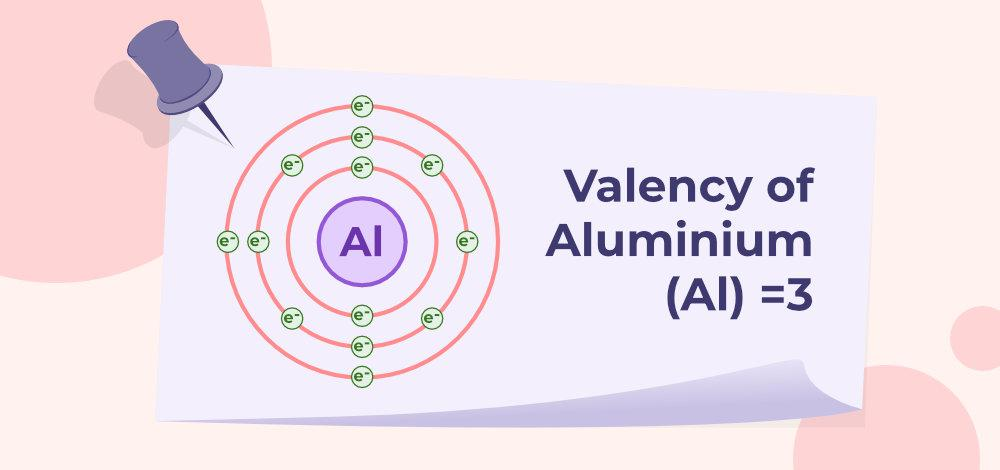### Valency of Potassium (K)

The Atomic number of K is 19 (K = 2; L = 8; M = 8, N=1). They have 1 electron in their outermost shell. So, the valency of Potassium is 1 as shown below: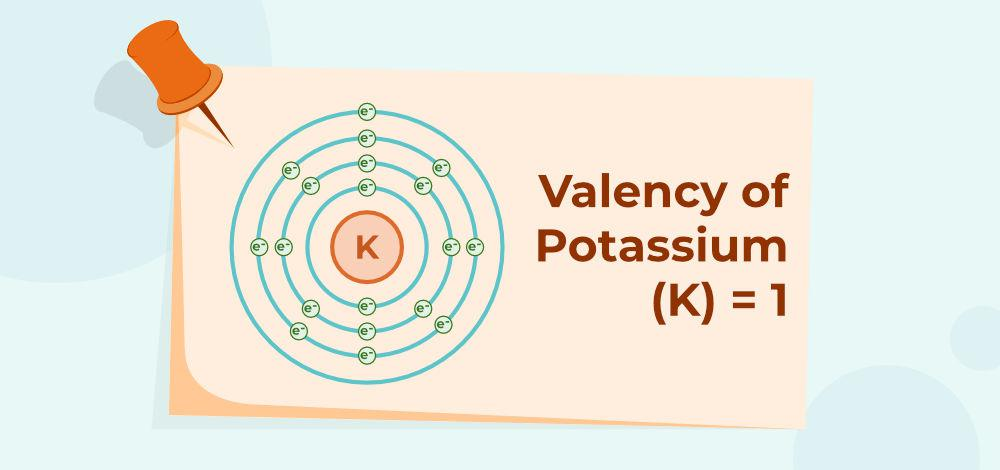### Valency of Magnesium (Mg)

The Atomic number of Mg is 12 (K = 2; L = 8; M = 2). They have 2 electrons in their outermost shell. So, the valency of Magnesium is 2 as shown below: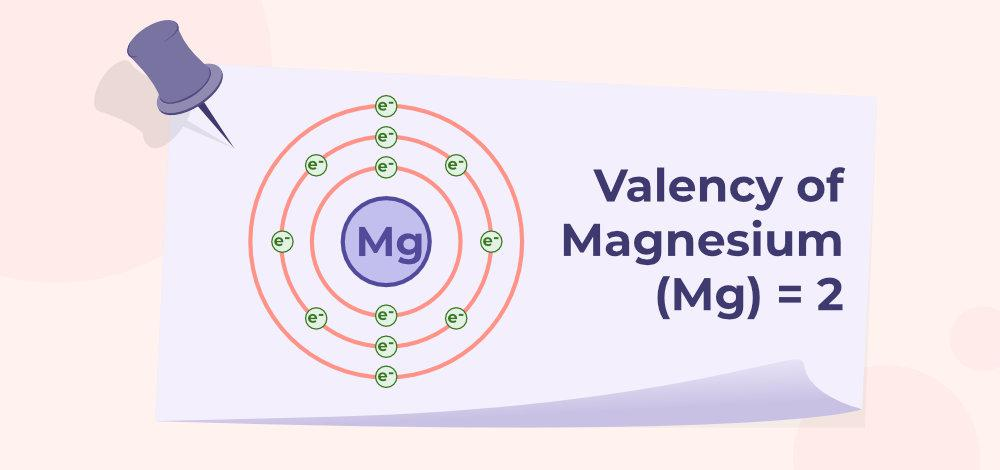## Valency Chart: Valency of First 20 elements

Here is the valency table which shows the valency of the first 20 elements from the periodic table with atomic numbers:

Read More: Atomic Number of Elements

## Valency Chart of Ions

There are many ions that show valency similar to elements, some of them as follows:

### Relation Between Valency and Valence Electrons

Valency explains the bond formation of atoms. Whereas, valence electrons are related to elemental characters. Valency is only a concept or idea and doesn’t involve the transmission of electrons. Whereas, valence electron involves the transmission of electrons in the formation of bonds. Both valency and valence electrons are applied to any chemical element.

Since only the valence electrons (outermost electrons) participate in chemical bonding, an element’s valency is determined by the number of valence electrons (outermost electrons) in its atom. The amount of valence electrons in an element’s atom or the number of electrons necessary to complete eight electrons in the valence shell determines the element’s valency. Sodium, for example, contains one valence electron and thus a valency of one. As a result, the valency of sodium is equal to the number of valence electrons in its atom. A metal element’s valency is equal to the number of valence electrons in its atom in general.

Valency of a Metal = Number of Valence Electrons in its Atom

A valence electron, like an electron in an inner shell, may receive or release energy in the form of a photon. Atomic excitation occurs when an electron gains enough energy to migrate (jump) to an outer shell. Ionization occurs when an electron breaks away from its associated atom’s valence shell, resulting in the formation of a positive ion. When an electron loses energy (and so emits a photon), it might migrate to an inner shell that isn’t completely occupied.

Valency of a Non-Metal = 8 – Number of Valence Electrons in its Atom

## FAQs on Valency

### Question 1: Define Valency.

The valency of an atom is equal to the number of valence electrons that this atom can gain or lose during chemical reactions. For example, the amount of hydrogen atoms, chlorine atoms, or double the number of oxygen atoms that one atom of an element may combine with is referred to as its valency.

### Question 2: What is the Valency of Chlorine?

The atomic number of Chlorine is 17, so its electronic configuration can be written 2, 8, 7. Therefore its valency is 1 only.

### Question 3: What is the Valency of Carbon?

The Electronic configuration of C is 6 (K = 2; L = 4). They have 4 electron in their outermost shell. So, the valency of Carbon is 4.

### Question 4: What is the Valency of Oxygen?

The Electronic configuration of O is 8 (K = 2; L = 6; M= 0), They have 6 electrons in their outermost shell. So, the valency of Oxygen is : 8 – 6 = 2.

### Question 5: How to Find the Valency of an Element?

The periodic table shows that all members of the same group of an element have the same valency. If we look at group 8 in the periodic table, all of the elements have achieved octet organisation and have entirely occupied their outermost orbit. Therefore, group 8’s elements have no valencies. There are three main ways to determine the valency of any element:

1. Using the complete octet rule,
2. Using the periodic table of elements, and
3. On the Basis of the Chemical Formulae.

### Question 6: Which of the following is an anion with valency two?

Chloride Ion, Ammonium Ion, Calcium Ion, Bromide Ion